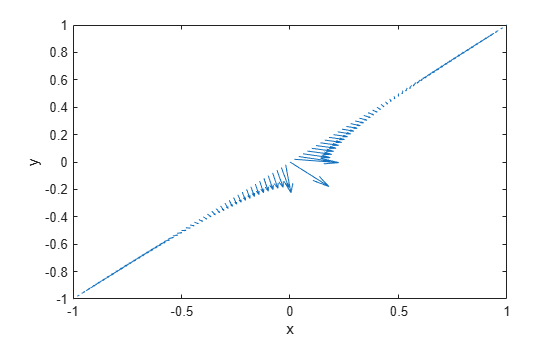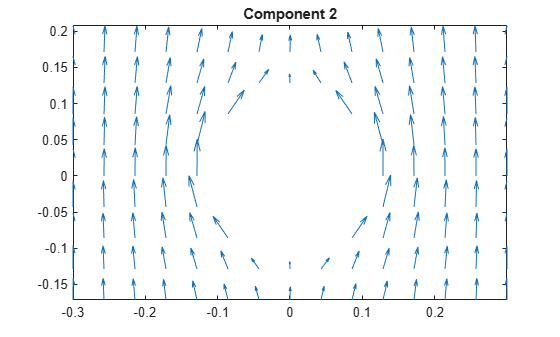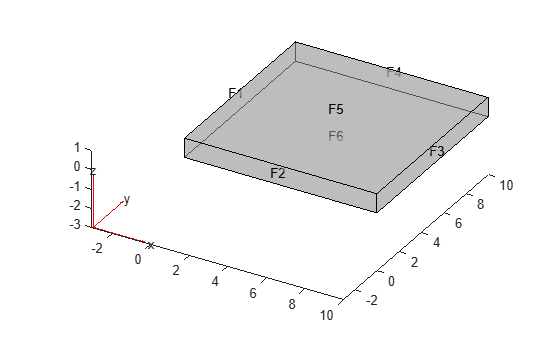Evaluate gradients of PDE solutions at arbitrary points

## Syntax

``````[gradx,grady] = evaluateGradient(results,xq,yq)``````
``````[gradx,grady,gradz] = evaluateGradient(results,xq,yq,zq)``````
``[___] = evaluateGradient(results,querypoints)``
``[___] = evaluateGradient(___,iU)``
``[___] = evaluateGradient(___,iT)``

## Description

example

``````[gradx,grady] = evaluateGradient(results,xq,yq)``` returns the interpolated values of gradients of the PDE solution `results` at the 2-D points specified in `xq` and `yq`.```

example

``````[gradx,grady,gradz] = evaluateGradient(results,xq,yq,zq)``` returns the interpolated gradients at the 3-D points specified in `xq`, `yq`, and `zq`.```

example

````[___] = evaluateGradient(results,querypoints)` returns the interpolated values of the gradients at the points specified in `querypoints`.```

example

````[___] = evaluateGradient(___,iU)` returns the interpolated values of the gradients for the system of equations for equation indices (components) `iU`. When solving a system of elliptic PDEs, specify `iU` after the input arguments in any of the previous syntaxes.The first dimension of `gradx`, `grady`, and, in 3-D case, `gradz` corresponds to query points. The second dimension corresponds to equation indices `iU`.```

example

````[___] = evaluateGradient(___,iT)` returns the interpolated values of the gradients for the time-dependent equation or system of time-dependent equations at times `iT`. When evaluating gradient for a time-dependent PDE, specify `iT` after the input arguments in any of the previous syntaxes. For a system of time-dependent equations, specify both time indices `iT` and equation indices (components) `iU`.The first dimension of `gradx`, `grady`, and, in 3-D case, `gradz` corresponds to query points. For a single time-dependent PDE, the second dimension corresponds to time-steps `iT`. For a system of time-dependent PDEs, the second dimension corresponds to equation indices `iU`, and the third dimension corresponds to time-steps `iT`.```

## Examples

collapse all

Evaluate gradients of the solution to a scalar elliptic problem along a line. Plot the results.

Create the solution to the problem $-\Delta u=1$ on the L-shaped membrane with zero Dirichlet boundary conditions.

```model = createpde; geometryFromEdges(model,@lshapeg); applyBoundaryCondition(model,'dirichlet', ... 'Edge',1:model.Geometry.NumEdges, ... 'u',0); specifyCoefficients(model,'m',0,... 'd',0,... 'c',1,... 'a',0,... 'f',1); generateMesh(model,'Hmax',0.05); results = solvepde(model);```

Evaluate gradients of the solution along the straight line from `(x,y)=(-1,-1)` to `(1,1)`. Plot the results as a quiver plot by using `quiver`.

```xq = linspace(-1,1,101); yq = xq; [gradx,grady] = evaluateGradient(results,xq,yq); gradx = reshape(gradx,size(xq)); grady = reshape(grady,size(yq)); quiver(xq,yq,gradx,grady) xlabel('x') ylabel('y')```Calculate gradients for the mean exit time of a Brownian particle from a region that contains absorbing (escape) boundaries and reflecting boundaries. Use the Poisson's equation with constant coefficients and 3-D rectangular block geometry to model this problem.

Create the solution for this problem.

```model = createpde; importGeometry(model,'Block.stl'); applyBoundaryCondition(model,'dirichlet', ... 'Face',[1,2,5], ... 'u',0); specifyCoefficients(model,'m',0,... 'd',0,... 'c',1,... 'a',0,... 'f',2); generateMesh(model); results = solvepde(model);```

Create a grid and interpolate gradients of the solution to the grid.

```[X,Y,Z] = meshgrid(1:16:100,1:6:20,1:7:50); [gradx,grady,gradz] = evaluateGradient(results,X,Y,Z);```

Reshape the gradients to the shape of the grid and plot the gradients.

```gradx = reshape(gradx,size(X)); grady = reshape(grady,size(Y)); gradz = reshape(gradz,size(Z)); quiver3(X,Y,Z,gradx,grady,gradz) axis equal xlabel('x') ylabel('y') zlabel('z')```Solve a scalar elliptic problem and interpolate gradients of the solution to a dense grid. Use a query matrix to specify the grid.

Create the solution to the problem $-\Delta u=1$ on the L-shaped membrane with zero Dirichlet boundary conditions.

```model = createpde; geometryFromEdges(model,@lshapeg); applyBoundaryCondition(model,'dirichlet', ... 'Edge',1:model.Geometry.NumEdges, ... 'u',0); specifyCoefficients(model,'m',0,... 'd',0,... 'c',1,... 'a',0,... 'f',1); generateMesh(model,'Hmax',0.05); results = solvepde(model);```

Interpolate gradients of the solution to the grid from -1 to 1 in each direction. Plot the result using the `quiver` plotting function.

```v = linspace(-1,1,101); [X,Y] = meshgrid(v); querypoints = [X(:),Y(:)]'; [gradx,grady] = evaluateGradient(results,querypoints); quiver(X(:),Y(:),gradx,grady) xlabel('x') ylabel('y')```Zoom in on a particular part of the plot to see more details. For example, limit the plotting range to 0.2 in each direction.

`axis([-0.2 0.2 -0.2 0.2])`Evaluate gradients of the solution to a two-component elliptic system and plot the results.

Create a PDE model for two components.

`model = createpde(2);`

Create the 2-D geometry as a rectangle with a circular hole in its center. For details about creating the geometry, see the example in Solve PDEs with Constant Boundary Conditions.

```R1 = [3,4,-0.3,0.3,0.3,-0.3,-0.3,-0.3,0.3,0.3]'; C1 = [1,0,0,0.1]'; C1 = [C1;zeros(length(R1)-length(C1),1)]; geom = [R1,C1]; ns = (char('R1','C1'))'; sf = 'R1 - C1'; g = decsg(geom,sf,ns);```

Include the geometry in the model and view the geometry.

```geometryFromEdges(model,g); pdegplot(model,'EdgeLabels','on') axis equal axis([-0.4,0.4,-0.4,0.4])```Set the boundary conditions and coefficients.

```specifyCoefficients(model,'m',0,... 'd',0,... 'c',1,... 'a',0,... 'f',[2; -2]); applyBoundaryCondition(model,'dirichlet', ... 'Edge',3,'u',[-1,1]); applyBoundaryCondition(model,'dirichlet', ... 'Edge',1,'u',[1,-1]); applyBoundaryCondition(model,'neumann', ... 'Edge',[2,4:8],'g',[0,0]);```

Create a mesh and solve the problem.

```generateMesh(model,'Hmax',0.1); results = solvepde(model);```

Interpolate the gradients of the solution to the grid from -0.3 to 0.3 in each direction for each of the two components.

```v = linspace(-0.3,0.3,15); [X,Y] = meshgrid(v); [gradx,grady] = evaluateGradient(results,X,Y,[1,2]);```

Plot the gradients for the first component.

```figure gradx1 = gradx(:,1); grady1 = grady(:,1); quiver(X(:),Y(:),gradx1,grady1) title('Component 1') axis equal xlim([-0.3,0.3])```Plot the gradients for the second component.

```figure gradx2 = gradx(:,2); grady2 = grady(:,2); quiver(X(:),Y(:),gradx2,grady2) title('Component 2') axis equal xlim([-0.3,0.3])```Solve a system of hyperbolic PDEs and evaluate gradients.

Import slab geometry for a 3-D problem with three solution components. Plot the geometry.

```model = createpde(3); importGeometry(model,'Plate10x10x1.stl'); pdegplot(model,'FaceLabels','on','FaceAlpha',0.5)```Set boundary conditions such that face 2 is fixed (zero deflection in any direction) and face 5 has a load of `1e3` in the positive `z`-direction. This load causes the slab to bend upward. Set the initial condition that the solution is zero, and its derivative with respect to time is also zero.

```applyBoundaryCondition(model,'dirichlet','Face',2,'u',[0,0,0]); applyBoundaryCondition(model,'neumann','Face',5,'g',[0,0,1e3]); setInitialConditions(model,0,0);```

Create PDE coefficients for the equations of linear elasticity. Set the material properties to be similar to those of steel. See Linear Elasticity Equations.

```E = 200e9; nu = 0.3; specifyCoefficients(model,'m',1,... 'd',0,... 'c',elasticityC3D(E,nu),... 'a',0,... 'f',[0;0;0]);```

Generate a mesh, setting `Hmax` to `1`.

`generateMesh(model,'Hmax',1);`

Solve the problem for times `0` through `5e-3` in steps of `1e-4`. You might have to wait a few minutes for the solution.

```tlist = 0:5e-4:5e-3; results = solvepde(model,tlist);```

Evaluate the gradients of the solution at fixed `x`- and `z`-coordinates in the centers of their ranges, 5 and 0.5 respectively. Evaluate for `y` from 0 through 10 in steps of 0.2. Obtain just component 3, the `z`-component.

```yy = 0:0.2:10; zz = 0.5*ones(size(yy)); xx = 10*zz; component = 3; [gradx,grady,gradz] = evaluateGradient(results,xx,yy,zz, ... component,1:length(tlist));```

The three projections of the gradients of the solution are 51-by-1-by-51 arrays. Use `squeeze` to remove the singleton dimension. Removing the singleton dimension transforms these arrays to 51-by-51 matrices which simplifies indexing into them.

```gradx = squeeze(gradx); grady = squeeze(grady); gradz = squeeze(gradz);```

Plot the interpolated gradient component `grady` along the `y` axis for the following six values from the time interval `tlist`.

```figure t = [1:2:11]; for i = t p(i) = plot(yy,grady(:,i),'DisplayName', ... strcat('t=',num2str(tlist(i)))); hold on end legend(p(t)) xlabel('y') ylabel('grady') ylim([-5e-6, 20e-6])```## Input Arguments

collapse all

PDE solution, specified as a `StationaryResults` object or a `TimeDependentResults` object. Create `results` using `solvepde` or `createPDEResults`.

Example: `results = solvepde(model)`

x-coordinate query points, specified as a real array. `evaluateGradient` evaluates the gradients of the solution at the 2-D coordinate points `[xq(i),yq(i)]` or at the 3-D coordinate points `[xq(i),yq(i),zq(i)]`. So `xq`, `yq`, and (if present) `zq` must have the same number of entries.

`evaluateGradient` converts query points to column vectors `xq(:)`, `yq(:)`, and (if present) `zq(:)`. For a single stationary PDE, the result consists of column vectors of the same size. To ensure that the dimensions of the gradient components are consistent with the dimensions of the original query points, use `reshape`. For example, use `gradx = reshape(gradx,size(xq))`.

For a time-dependent PDE or a system of PDEs, the first dimension of the resulting arrays corresponds to spatial points specified by the column vectors `xq(:)`, `yq(:)`, and (if present) `zq(:)`.

Data Types: `double`

y-coordinate query points, specified as a real array. `evaluateGradient` evaluates the gradients of the solution at the 2-D coordinate points `[xq(i),yq(i)]` or at the 3-D coordinate points `[xq(i),yq(i),zq(i)]`. So `xq`, `yq`, and (if present) `zq` must have the same number of entries.

`evaluateGradient` converts query points to column vectors `xq(:)`, `yq(:)`, and (if present) `zq(:)`. For a single stationary PDE, the result consists of column vectors of the same size. To ensure that the dimensions of the gradient components are consistent with the dimensions of the original query points, use `reshape`. For example, use `grady = reshape(grady,size(yq))`.

For a time-dependent PDE or a system of PDEs, the first dimension of the resulting arrays corresponds to spatial points specified by the column vectors `xq(:)`, `yq(:)`, and (if present) `zq(:)`.

Data Types: `double`

z-coordinate query points, specified as a real array. `evaluateGradient` evaluates the gradients of the solution at the 3-D coordinate points `[xq(i),yq(i),zq(i)]`. So `xq`, `yq`, and `zq` must have the same number of entries.

`evaluateGradient` converts query points to column vectors `xq(:)`, `yq(:)`, and (if present) `zq(:)`. For a single stationary PDE, the result consists of column vectors of the same size. To ensure that the dimensions of the gradient components are consistent with the dimensions of the original query points, use `reshape`. For example, use `gradz = reshape(gradz,size(zq))`.

For a time-dependent PDE or a system of PDEs, the first dimension of the resulting arrays corresponds to spatial points specified by the column vectors `xq(:)`, `yq(:)`, and (if present) `zq(:)`.

Data Types: `double`

Query points, specified as a real matrix with either two rows for 2-D geometry, or three rows for 3-D geometry. `evaluateGradient` evaluates the gradients of the solution at the coordinate points `querypoints(:,i)`, so each column of `querypoints` contains exactly one 2-D or 3-D query point.

Example: For 2-D geometry, ```querypoints = [0.5,0.5,0.75,0.75; 1,2,0,0.5]```

Data Types: `double`

Equation indices, specified as a vector of positive integers. Each entry in `iU` specifies an equation index.

Example: `iU = [1,5]` specifies the indices for the first and fifth equations.

Data Types: `double`

Time indices, specified as a vector of positive integers. Each entry in `iT` specifies a time index.

Example: `iT = 1:5:21` specifies every fifth time-step up to 21.

Data Types: `double`

## Output Arguments

collapse all

x-component of the gradient, returned as an array. For query points that are outside the geometry, `gradx` = `NaN`. For information about the size of `gradx`, see Dimensions of Solutions, Gradients, and Fluxes.

y-component of the gradient, returned as an array. For query points that are outside the geometry, `grady` = `NaN`. For information about the size of `grady`, see Dimensions of Solutions, Gradients, and Fluxes.

z-component of the gradient, returned as an array. For query points that are outside the geometry, `gradz` = `NaN`. For information about the size of `gradz`, see Dimensions of Solutions, Gradients, and Fluxes.

## Tips

The `results` object contains the solution and its gradient calculated at the nodal points of the triangular or tetrahedral mesh. You can access the solution and three components of the gradient at nodal points by using dot notation.

`interpolateSolution` and `evaluateGradient` let you interpolate the solution and its gradient to a custom grid, for example, specified by `meshgrid`.

## Version History

Introduced in R2016a